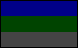This document is part of the Martian Time Boneyard. It was originally located at http://www.geocities.com/sotosoroto/bumpy.html. Author: Mark KnokeCurved Monthly Perpetual Calendar Ideas

Mark Knoke

By 'curved', I mean that these calendars try to fit the changing lengths of the Martian seasons. Vertum (northern spring) has 193 days, Sumwin (northern summer) has 179 days, Tumver (northern autumn) has 143 days, and Winsum (northern winter) has 154 days. These season lengths change by a couple minutes each year, but that's only a day difference over a thousand years, so these calendars won't be out of date anytime soon. By 'monthly perpetual', I mean that each month has a whole number of weeks in it. Intercalary days could be lumped together in short weeks called 'weks'. These funky naming ideas are Peter Kokh's, actually, but he wasn't completely serious about wek...

6-day weeks, 22 or 19 months

4x30+2x36=192, 1 short
6x30=180, 1 long
4x30+1x24=144, 1 long
5x30=150, 4 short
=22x../36=666

Off by a total of 4 days when you add one 'wek' at then end of Winsum. Although there are 3 month lengths, the two long one are the longest season and the short one is in the shortest season. So it can be rationalized, eh?

Another possibility:
4x30+2x36=192
6x30=180
4x36=144
5x30=150
=19x30/36=666

7-day weeks, 20 months

4x35=140, 3 short
2x35+3x28=154, exact
2x28+4x35=196, 3 long
5x35=175, 4 short
=20x28/35=665

with 4 extra days to kick around, it's off by a total of 6 days -- not quite as accurate as the other versions.

8-day weeks, 22 or 18 months

6x32=192, 1 short
4x32+2x24=176, 3 short
3x32+2x24=144, 1 long
4x32+1x24=152, 2 short
=22x24/32=664

spread the 5 extras amongst the short's, and you're only off by a total of 2 days! But of course you can't clump your short months together like you could in others. Who knows? Maybe that's a good thing.

Another possibility:
1x32+4x40=192
3x32+2x40=176
2x32+2x40=144
1x42+3x40=152
=18x32/40=664

9-day weeks, 20 months

4x36=144, 1 long
3x27+2x36=153, 1 short
3x27+3x36=189, 4 short
5x36=180, 1 long
=20x27/36=666

Add the three extra days to Vertum, and they're only off by a total of 4 days.

10-day weeks, 21 months

5x30+1x40=190, 3 short
6x30=180, 1 long
2x30+2x40=140, 3 short
5x30=150, 4 short
=21x30/40=660

also a total of 2 days off, if you don't mind a few 'weks' per year.

11-day weeks, 18 months

3x33+2x44=187, 6 short
4x33+1x44=176, 3 short
3x33+1x44=143, exact
2x33+2x44=156, 2 long
=18x33/44=660

similarly 2 days off with a couple 'weks'.

Oh my, six through eleven, a bit more than I intended to. ...Pick your favorite and make it better!. . | . .. . | . .to my homepage . | . to my Mars page . | . to my Narakan page